# Study The Shift In Equilibrium Between Ferric Ions And Thiocyanate Ions By Increasing/Decreasing The Concentration Of Either Of The Ions

Let us study the equilibrium reaction between potassium thiocyanate and ferric chloride through the change in the concentration of colour of the solution. The reaction and equilibrium constant is given by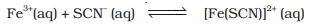The equilibrium constant is given by the formula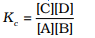Finding equilibrium constant for the reaction between potassium thiocyanate and ferric chloride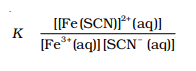At a constant temperature, the value of K also remains constant.

Increasing the concentration of either Fe3+ ion or thiocyanate ion would result in an increase in the concentration of [Fe(SCN)]2+ ions.

## Aim:

To understand the process of shift in equilibrium between ferric ions and thiocyanate ions by either increasing/decreasing the concentration of the ions.

## Materials Required:

0.100g Ferric chloride, 0.100g Potassium thiocyanate, 2 Beakers of 100 mL capacity, 250 mL Beaker, 6 Boiling tubes, 4 Burettes, 2 Glass droppers, 1 Test tube stand, 1 Glass rod

## Experimental setup: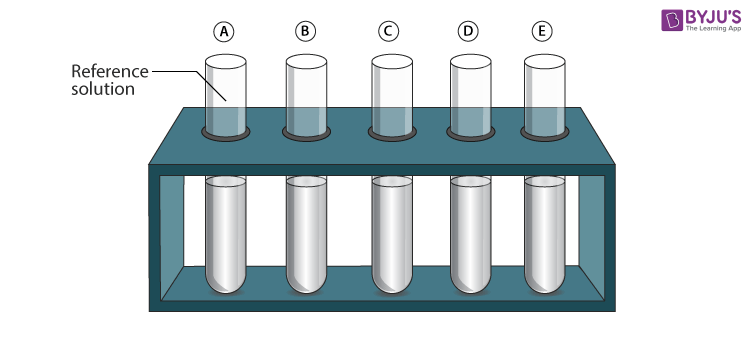## Procedure:

1. In a beaker, dissolve 0.100g of ferric chloride salt in 100 mL of water and in another beaker, dissolve 0.100 g potassium thiocyanate in 100 mL of water.
2. By mixing 20 mL of ferric chloride solution with 20 mL of potassium thiocyanate solution, a bright blood red colour solution will be obtained.
3. Fill this bright blood red colour solution in a burette.
4. Take five boiling tubes measuring of equal size and then label them as a,b,c, d, and e.
5. Add 2.5 mL of blood red solution to each of the boiling tubes from the burette.
6. Add 17.5 mL of water to the boiling tube ‘a’, so that the total volume of solution in the boiling tube ‘a’ is 20 mL. (for your reference)
7. Now take three burettes and label them as A, B, and C.
8. Fill ferric chloride solution in burette A
9. FIll potassium thiocyanate solution in burette B
10. Fill burette C with water
11. Add 1.0 mL, 2.0 mL, 3.0 mL and 4.0 mL of ferric chloride solution to boiling tubes b, c, d, and e respectively from burette A.
12. From burette C add 16.5 mL, 15.5 mL, 14.5 mL, and 13.5 mL of water to boiling tubes b, c, d, and e respectively.
13. Compare the colour intensity produced from the solution in each boiling tube with the colour of the reference solution in the boiling tube ‘a’.
14. Take another set of four clean boiling tubes and fill them with 2.5 mL of blood red solution to each of the boiling tubes from the burette.
15. Repeat the experiment by adding 1.0 mL, 2.0 mL, 3.0 mL and 4.0 mL of potassium thiocyanate solution from burette B to the boiling tubes b′, c′, d′, and e′ respectively followed by addition of 16.5 mL, 15.5 mL, 14.5 mL and 13.5 mL of water.
16. Again compare the colour intensity of the solution of these test tubes with reference equilibrium solution in the boiling tube ‘a’.
17. Record your results in the table given below
18. You may repeat the observations with different amounts of potassium thiocyanate and ferric chloride solution and compare with the reference solution.

## Precautions to be taken during the experiment:

• Use mildly diluted solutions of ferric chloride and potassium thiocyanate.
• Keep a watch on the colour of the solutions in the boiling tube and reference test tube
• Use boiling tubes of the same size.

## Observation

Study of Equilibrium shift when the concentration of ferric ions is increased

 Boiling Tube Amount of ferric chloride solution Change in colour intensity as matched with the reference solution in tube “a” The direction of shift in equilibrium a Reference solution 2.5 mL blood red solution + 17.5 mL water Equilibrium position b 1.0 c 2.0 d 3.0 e 4.0

Study of Equilibrium shift when the concentration of thiocyanate ions are increased

 Boiling Tube The volume of thiocyanate Change in colour intensity as matched with the reference solution in tube “a” The direction of shift in equilibrium a Reference solution 2.5 mL blood red solution + 17.5 mL water Equilibrium position b 1.0 c 2.0 d 3.0 e 4.0

## Viva Voice:

1. Does the constancy in colour intensity indicate the dynamic nature of equilibrium? Explain your answer with appropriate reasons.No, since the colour becomes constant even after the reaction stops at equilibrium.
2. What is equilibrium constant and how does it differ from the rate constant?The equilibrium constant is given by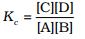The equilibrium constant is independent of the initial concentration of reactants and is a function of temperature but remains constant at a constant temperature.
3. Why is it suggested to carry the experiment with dilute solutions?Dilute solutions are safer on heating and do not cause damage in lab conditions.
4. Does temperature affect equilibrium?Yes
5. Why are the boiling tubes of the same size used in the experiment’?
To get the exact measurement of the solution added for reference.

Take up a quiz on Shift In Equilibrium Between Ferric Ions And Thiocyanate Ions